# The Butterfly Theorem

According to Coxeter and Greitzer, one of the solutions to the Butterfly theorem was submitted in 1815 by W. G. Horner of Horner's method fame. Most recently a 1805 proof by William Wallace has been discovered in Wallace's family archives. Still, later it was found that Wallace's posting of a more general problem dates to 1803.

The solutions I gathered below may serve as a basis for a discussion of which proofs better clarify the gist of the problem, if at all. Why is the result true? (See the discussions on Concyclic Circumcenters: Dynamic View and A Sequel.)

### TheoremLet M be the midpoint of a chord PQ of a circle, through which two other chords AB and CD are drawn; AD cuts PQ at X and BC cuts PQ at Y. Prove that M is also the midpoint of XY.

### Proof 0 (William Wallace, 1805)

William Wallace's proof is based on the following diagram:I have placed it on a separate page.### Proof 1 [Coxeter and Greitzer, p. 45]

Let's drop perpendiculars x1 and x2 from X and y1 and y2 from Y on AB and CD. Let's also introduce a = MP = MQ and x = XM and y = YM. Observe several pairs of similar triangles, which implies the following proportions:

Triangles Proportion Mx1 and My1 x/y = x1/y1 Mx2 and My2 x/y = x2/y2 Ax1 and Cy2 x1/y2 = AX/CY Dx2 and By1 x2/y1 = XD/YB

from which

x²/y² = x1/y2· x2/y1 = x1/y1· x2/y2 = AX·XD/ CY·YB = PX·XQ/ PY·YQ.

So that

x²/y² = (a - x)(a + x)/ (a - y)(a + y) = (a² - x²)/(a² - y²) = a²/a² = 1.

And finally x = y.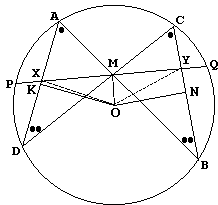### Proof 2 [Shklyarsky, problem 104, solution 1]

Let O be the center of the given circle. Since OM ⊥ XY, in order to show that XM = MY, we have to prove that ∠XOM = ∠YOM.

Drop perpendiculars OK and ON from O onto AD and BC, respectively. Obviously, K is the midpoint of AD and N is the midpoint of BC. Further,

∠DAB = ∠DCB and ∠ADC = ∠ABC,

as angles subtending equal arcs. Triangles ADM and CBM are therefore similar, and AD/AM = BC/CM, or AK/AM = CN/CM. In other words, in triangles AKM and CNM two pairs of sides are proportional. Also, the angles between the corresponding sides are equal. We infer that the triangles AKM and CNM are similar. Hence, ∠AKM = ∠CNM.

Now, have a look at the quadrilaterals OKXM and ONYM. Both have a pair of opposite straight angles, which implies that both are inscribable in a circle. In OKXM, ∠AKM = ∠XOM. In ONYM, ∠CNM = ∠YOM. From which we get what we've been looking for: ∠XOM = ∠YOM.### Proof 3 [Shklyarsky, problem 104, solution 2]

For convenience, denote the angles as on the diagram on the right. Let x = XM and a = PM. As in Proof 1,

 AX·XD = PX·XQ = a² - x².

In ΔDXM, by the Law of Sines, we have

 DX; = x·sin(α)/sin(180° - (α + β + γ)) = x·sin(α)/sin(α + β + γ).

And in ΔAXM

AX = x·sin(β)/sin(γ),

AX·DX = x²·sin(α)·sin(β)/sin(γ)·sin(α + β + γ) = a² - x².

From here we may find x²:

x² = a²·sin(γ)·sin(α + β + γ))/(sin(α)·sin(β) + sin(γ)·sin(α + β + γ)).

The latter expression is symmetric in α and β. Therefore, if we repeat the derivation for the segment y = MY, we'll get exactly same result. Hence, x = y.### Proof 4

This proof originates in Projective geometry, but could be explained without invoking the theory. (It's loosely based on problem 29.46 from [Problems].) Let S be the given circle with center O. Assume for a moment that there exists a (projective) transformation T of the plane that

1. maps S onto another circle S',
2. moves M onto the center O' of S',
3. preserves the ratio of segments in the direction perpendicular to OM,
4. transforms AB, CD, AD, BC, and PQ onto the segments A'B', C'D', A'D', B'C', and P'Q' with
5. P'Q' still perpendicular to OM.

(I'll prove the existence of T shortly.) A'B', C'D' and P'Q' serve as diameters of S'. O' is the center of a half-turn that takes A' into B', C' into D', P' into Q', and, therefore, X' into Y', where X' and Y' are images of X and Y. Triangles A'X'O' and B'Y'O' are then equal, so that X'O' = O'Y'. But, by #3 and #5 above, XM/MY = X'O'/O'Y'. Therefore, too, XM = MY.

It remains to be shown that the transformation T with properties 1-5 does exist. I'll construct T as a composition of two transformations: a central projection and an affine mapping.Let UV be the diameter of S through M. UV ⊥ PQ. Imagine looking at the circle from a side on the level of its plane in the direction of PQ. We see a segment UV with the midpoint at O and another point M between O and U. Choose a point K on a perpendicular to UV at O. Draw KM. Find a line VNW such that VN = NW. (This is simple: just draw a line through O parallel to KU. Let N be the point of intersection of that line with AM. Extend VN to the intersection with AU at W.)

Now, think of K as the apex of a right cone with base S. VW is then an axis of the ellipse cut from the cone by a plane through VW perpendicular to the plane of the drawing. N is its center. The central projection I mentioned above maps M to N, U to W, V to V, and, in general, a point Z in the plane of S to the point of intersection of KZ with the plane of the ellipse. The latter could now be squeezed in the direction of VW so as to map the ellipse onto a circle keeping N as its center.

It's quite obvious that the composition of the two transformations satisfies 1-5 above.### Proof 5 [Essays, p. 59]

The proof is very simple but requires familiarity with a general notion of conics and second degree equations that describe the conics in Cartesian coordinates.

In the plane Cartesian coordinates conics are described by second degree equations:

f(x, y) = Ax² + Bxy + Cy² + Dx + Ey + F = 0.

Any (non zero) multiple of such an equation, describes exactly the same conic, which is to say that it takes 5 numbers to describe a conic, not 6 as might appear from the equation. A conic is thus uniquely determined by 5 distinct points.

Straight lines are described by linear equations

l(x, y) = Ax + By + C = 0.

Straight lines are uniquely determined by two points. Below, for a point X with coordinates (x, y), l(x, y) and l(X) are used interchangeably, depending on which is more convenient under the circumstances. With these preliminaries we may prove the following

### Lemma

Let the points P, Q, R, and S lie on a conic given by a second degree equation f = 0. Then, for some constant λ and μ,

f = λ·lPQlRS + μ·lPSlQR,

where lPQ = 0, lRS = 0, lPS = 0, and lQR = 0 are the (linear) equations of PQ, RS, PS and QR, respectively.

### Proof of Lemma

Let U be a fifth point on the conic at hand. Then surely there exist λ0 and μ0 such that

λ0·lPQ(U)·lRS(U) + μ0·lPS(U)·lQR(U) = 0.

Define a second degree expression

f0(X) = λ0·lPQ(X)·lRS(X) + μ0·lPS(X)·lQR(X).

Clearly f0(U) = 0. But also f0(P) = f0(Q) = f0(R) = f0(S) = 0. (For example, f0(P) = 0 because lPQ(P) = 0 and lPS(P) = 0.) The equation f0(X) = 0 defines the same conic as f = 0, so that f and f0 are multiples of each other: f = αf0 for some α. Which proves the lemma.Let's now continue the proof of the Butterfly theorem. Let f = 0 be an equation of the given circle. Then by lemma, for some λ and μ

This equation also holds for the restrictions of all the functions involved onto the line PQ. Take PQ as the x-axis with the origin at M. Then, since f(P) = f(Q) = 0 and PM = MQ, we can assume that f(x, 0) = x² - a and lABlCD = bx² on PQ. If so, restricted to PQ, lADlBC = cx² - d. This means that the two roots of the equation lADlBC = 0 are equidistant from the origin M.### Proof 5'

The Butterfly theorem was offered as problem A6 at the 24th Putnam competition (1963). The solution I came across on the Web, is a modification of Proof 5 based on a slightly more general lemma: Let g = 0 and h = 0 be equations of two distinct conics through 4 distinct points. Then any conic through the four points has the equation λg + μh = 0, for some λ and μ.

The circle is then defined, by, say, g = 0 and the pair of lines AB, CD by h = 0. Then the pair of lines AD, BC is another conic through the same four points and satisfies f = λg + μh = 0, for some λ and μ. Now, g and h have symmetric (with respect to M) restrictions to PQ, and so has f.

### RemarkNote that the latter proof only requires that the restrictions of AB and CD on PQ be symmetric with respect to M. This means that the equality PX = QY holds not only when AB and CD pass through M, but also when they cross PQ at points equidistant from M! We thus get a simple proof of the generalization of the Butterfly theorem first established by M. Klamkin in 1965 (Mathematics Magazine, Volume 38, Issue 4 (Sep., 1965), 206-208.)### Proof 6

The Butterfly theorem is a particular case of a more general Two Butterflies theorem. Choose one "butterfly quadrilateral" to be symmetric with respect to OM and so that its intersecting sides meet at M. The second "butterfly quadrilateral" is bound to intersect PQ at the same points as the first, i.e., symmetrically with respect to M.### Proof 7

Another generalization of the theorem was pointed out to me by Qiu FaWen, a Chinese teacher who discovered the result with his students in 1997. The Chinese version is no longer available. Any way, I had to do some guess work to figure out what it was about.

The Butterfly theorem follows trivially from the generalization when the two circles involved coalesce into one.### Proof 8

This proof is by Steve Conrad as appeared in Samuel Greitzer's Arbelos.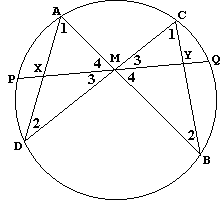The basic point of departure is the observation that, if in two triangles an angle of one is equal to an angle of the other, then the areas of the triangles are in the same ratio as the products of the sides composing the equal angles. In the diagram above, there are four pairs of equal angles, which allows us to write four equalities:

 1: Area(XAM)/Area(MCY) = AX·AM / CM·CY 2: Area(CMY)/Area(DMX) = CM·MY / DM·MX 3: Area(XDM)/Area(MBY) = DX·DM / BM·BY 4: Area(BMY)/Area(AMX) = BM·MY / AM·MX

Now, since the product of the ratios on the left equals 1, the same is true for the product of the right hand sides, which after some cancellation appears as

AX·DX·MY² / CY·BY·MX² = 1,

or

(1)

AX·DX / CY·BY = MX²/ MY².

By the power of a point theorem,

AX·DX = PX·QX = (MP - MX)·(MQ + MX)

and similarly

CY·BY = QY·PY = (MQ - MY)·(MP + MY).

Hence, from (1)

(2)

(MP - MX)·(MQ + MX) / MX² = (MQ - MY)·(MP + MY)/ MY².

Since MP = MQ, we obtain

MP²/ MX² - 1 = MP²/ MY² - 1,

which implies MX = MY.

### Remark

Without the assumption MP = MQ, (2) simplifies to

MP·MQ·(MX - MY) = MX·MY·(MP - MQ).

The latter is the essence of A. Candy's theorem (1896), which is usually written as

1/MP - 1/MQ = 1/MX - 1/MY.

As it turns out, this is very close to Candy's original derivation.### Proof 9

Following is a proof of Klamkin's generalization with the aid of Menelaus' theorem.Triangle XSY is cut by two transversals, CZD and AWB such that we may apply Menelaus' theorem twice:

 (AWB) XA/SA · SB/YB · YW/XW = 1 (CZD) XD/SD · SC/YC · YZ/XZ = 1

The product of the two is

(3)

XA·XD/SA·SD · SB·SC/YB·YC · YZ/XZ · YW/XW = 1.

From the Power of the Point theorem, SA·SD = SB·SC, so that (3) simplifies to

(4)

XA·XD/YB·YC · YZ/XZ · YW/XW = 1.

By the same theorem XA·XD = PX·QX and YB·YC = PY·QY. Therefore (4) becomes

(5)

PX·QX/PY·QY · YZ/XZ · YW/XW = 1.

Let PM = MQ = a, ZM = MW = b, XM = x, YM = y, and rewrite (5) as

(a - x)·(a + x)/(a + y)·(a - y) · (y + b)/(x - b) · (y - b)/(x + b) = 1.

which is just

(a² - x²)/(a² - y²) · (b² - y²)/(b² - x²) = 1,

or

(a² - x²)/(a² - y²) = (b² - x²)/(b² - y²).

The latter is equivalent to

(a² - b²)·(x² - y²) = 0.

Finally, except for the degenerate case of a = b where the chord becomes the tangent, we get x = y. The latter is of course also true when a = b, i.e. when all the points on PQ coalesce into one -- M.### Proof 10

I shall again prove Klamkin's generalization.

Consider two pencils of lines, one through A, the other through C. Relate those lines from the two pencils that intersect on the given circle, such that AP corresponds CP, AD to CD, AB, to CB, and AQ to CQ. All the pertinent angles between the pairs of related lines are equal as subtending the same arc. Therefore, A(PDBQ) = C(PDBQ). By the properties of cross-ratio, A(PDBQ) = (PXWQ), whereas C(PDBQ) = (PZYQ), which gives

(PXWQ) = (PZYQ).

(6)

WP/WX : QP/QX = YP/YZ : QP/QZ.

But since ZM = MW, we also have WP = QZ, so that we can rewrite (6) as

(6')

QX/WX = YP/YZ : QP/QZ.

Using the convention of the previous proof, (6') becomes

(a + x)/(b + x) = (a + y)/(b + y),

which is equivalent to (a - b)(x - y) = 0. Since a > b, the only possibility is x = y.### Proof 11

The proof is due to Professor Hiroshi Haruki [Honsberger and Diamonds, 136-138] and depends on his wonderful lemma, which we apply to the diagram below:Think of A and C as being two positions of a variable point traversing the circle. Then Haruki's lemma leads to

XP·MQ / XM = MP·YQ / YM,

which, because of MP = MQ, is simplified to

XP / XM = YQ / YM.

Adding 1 to both sides gives

(XP + XM) / XM = (YQ + YM) / YM.

Applying MP = MQ again, we obtain the required XM = YM.

Note that with a little change in the above we can easily obtain Morrey Klamkin's generalization.

### Proof 12

I have borrowed this proof from Paris Pamfilos' web site which since disappeared or moved. The proof also appears in H. Eves's A Survey of Geometry (pp. 144-145).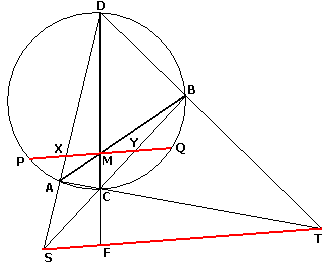Let S and T be the points of intersection of AD, BC and AC, BD. Then ST is the polar of M with respect to the given circle. In particular, this means that, if O is the center of the circle, then OM is perpendicular to ST. Since M is the middle of PQ, PQ, too, is perpendicular to OM. Hence, ST||PQ.

Further, from the construction via the complete quadrilateral of pairs of conjugate points, it follows that the conjugate (F) of M with respect to the pair C, D lies on ST. As a consequence, the lines SD, SC, SM, SF form a harmonic bundle. Any line crosses such a bundle in conjugate points. In particular, PQ crosses it in points X, Y, M, and infinity (recall that ST||PQ), which me know implies that MX = MY.

### Remark

Paris' proof actually shows more. For M not necessarily the midpoint of PQ, PQ will cross ST at some point N say. As before there are several harmonically conjugate pairs. In particular, (XYMN) = -1 and, of course, (PQMN) = -1. For these two,

1/XM + 1/YM = 2/NM, and
1/PM + 1/QM = 2/NM,

where all segments are signed. Subtracting and reversing to regular lengths, we get Candy's generalization, because, as signed segments, XM and YM and also PM and QM have different signs.

### Proof 13

This one comes from the first volume of the encyclopedic Problems in Planimetry by V. V. Prasolov (#2.54). We shall be proving Klamkin's generalization.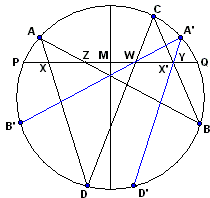Reflect points A, B, D and X in the perpendicular to PQ through its midpoint M. Denote the reflections A', B', D', and X'. A', B', D' lie on the given circle, whereas X' lies on PQ. We wish to show that X' coincides with Y.

Since Z and W are symmetric in M, A'B' passes through W. Further, we may observe the equality of several directed (or signed) angles between pairs of straight lines:

 ∠(WY, CY) = ∠(B'B, CB) (BB'||PQ) ∠(B'B, CB) = ∠(B'A', CA') (subtended by arc B'C) ∠(B'A', CA') = ∠(WA', CA') (A'B' passes through W)

Hence quadrilateral WYA'C is cyclic. We have an additional chain of equalities among angles:

 ∠(WX', A'X') = ∠(DD', A'D') (DD'||PQ) ∠(DD', A'D') = ∠(DC, A'C) (subtended by same arc) ∠(DC, A'C) = ∠(WC, A'C) (W lies on CD)

Hence quadrilateral WX'A'C is also cyclic and has exactly same circumcircle as WYA'C. Since, besides W, PQ intersects this circle in at most one other point, Y = X'. Q.E.D.

### Proof 14

While pursuing a delightful proof by Professor Haruki, I have first overlooked what R. Honsberger calls a standard proof [Honsberger and Diamonds, 135-136]We prove the original problem, so that M is the center of the chord PQ which is thus perpendicular to the diameter MO. Let B' be the reflection of B in that diameter. In particular, BB' is also perpendicular to OM and is divided by OM into equal parts. ΔBB'M is isosceles and its base angles 1 and 2 are equal. Further, BB'||PQ. Angle 3 is alternate with 1 and angle 4 with 2. It follows that all four are equal.

In a cyclic quadrilateral ABB'D angles at B (2) and D (5) are supplementary. They add up to 180°. If so, angles 3 and 5 also add up to 180°, which makes quadrilateral MXDB' cyclic. In this quadrilateral angles 6 and 7 subtend the same chord MX. They are thus equal. For the same reason, but in the given circle, angles 7 and 8 are equal. Which shows that angles 6 and 8 are also equal.

Now consider triangles MBY and MB'X. They have equal sides MB and MB', and equal pairs of adjacent angles: 3/4 and 6/8. By SAS, the triangles are equal. Therefore, MX = MY.

### Proof 15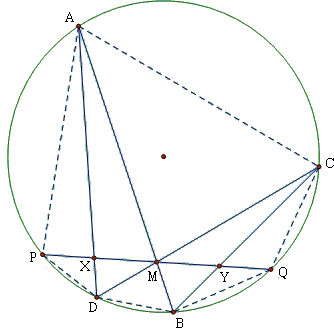This proof is by weininjieda from Yingkou, China who plans to become a teacher of mathematics, Chinese and history. As in proof #8, weininjieda compares areas of several triangles based, in particular, on the observation related to cyclic quadrilateral. For example, quadrilateral ACDP is split by the diagonal AD into two triangles, APD and ACD whose opposing angles at P and C add up to 180°. Thus,

Area(ΔAPD)/Area(ΔACD) = AP·DP / AC·CD.

Denote, as before, a = MP = MQ, x = MX, y = MY. Then

 PX/MX × MY/QY = (a - x)/x · y/(a - y) = Area(ΔAPD)/Area(ΔAMD) × Area(ΔCMB)/Area(ΔCQB) = Area(ΔAPD)/Area(ΔACD) × Area(ΔCAD)/Area(ΔCBD)  × Area(ΔBDC)/Area(ΔBQC) × Area(ΔBMC)/Area(ΔAMD) = AP·DP/AC·CD × AD·AC/BC·BD × BD·CD/BQ·CQ × BM·CM/AM·DM = AP·DP·AD·BM·CM / BC·BQ·CQ·AM·DM = DP/CQ × AP/BQ × AD/BC × BM/DM × CM/AM = MP/CM × AM/MQ × DM/BM × BM/DM × CM/AM = MP/MQ = 1.

It follows that (a - x)/x = (a - y)/y, or a/x - 1 = a/y - 1 so that x = y.

### Proof 16

This proof is by Greg Markowsky.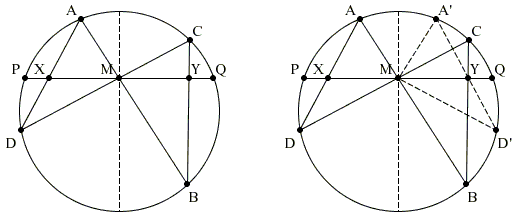Since M is the midpoint of PQ, the diameter of the circle through M is obviously perpendicular to PQ. Reflect A and D in that diameter to obtain points A' and D'. Observe that points A' and B form a pair of reflection about M; and so are points C and D'. By Greg's theorem about two such pairs, lines A'D' and BC meet on PQ, i.e., at Y. Since A'D' is the reflection of AD in the diameter through M, MX = MY.

### Proof 17

The proof has been communicated to me by Giles Gardam, a member of Australia's 2007 and 2008 IMO teams, and is due to his mentor, Ivan Guo, who won a gold medal at IMO 2004.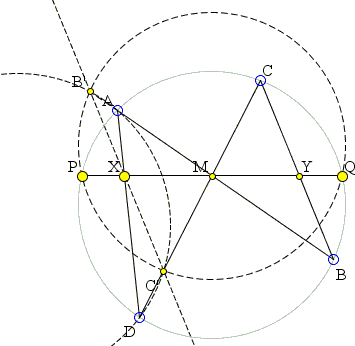An interactive illustration is available elsewhere.

Reflect B and C and the circle T in M (by reflect in a point I mean a dilation by factor -1 about that point). B', C', and T' be the reflections of B, C and circle T, respectively. Note that as M is the midpoint of PQ, T' passes through P and Q.

By power of point M with respect to T, AM×BM=CM×DM.

Thus AM×B'M=C'M×DM, so by converse of power of a point, AB'C'D is a cyclic quadrilateral. Let its circumcircle be T''.

We now consider the three radical axes of the three circles, which are PQ, AD and B'C', and concur by the radical axes theorem. Thus B'C' intersects PQ at X. The reflections of BC and PQ are B'C' and PQ, so considering their intersections, we have that X is the reflection of Y, thus M is the midpoint of XY.

### Proof 18

This proof is by B. Elsner alias MathOMan. (Check his link to an amazing video of Professor Jean-Pierre Kahane engaging passers-by in the street, using the butterfly theorem as one of the attractions.)For a plain analytic proof, assume for simplicity that the radius of the circle is 1, PQ lies on the horizontal x-axis, with the origin at M and the center of the circle O at (0, m), |m| < 1. The equation of the circle then is x² + (y - m)² = 1 and that of PQ is y = 0.

We may assume that neither AB nor CD coincide with PQ. Since both path through the origin, their equations are, say, x = ay, for AB, and x = by, for CD. Observe that in this form AB and CD are allowed to be vertical. We may assume a ≠ b as otherwise the problem does not make much sense.

Substituting x = ay in the equation of the circle, we see that the ordinates of the points A(xA, yA) and B(xB, yB) satisfy the equation a²y² + (y - m)² = 1, implying

(1 + a²)y² - 2my + (m² - 1) = (1 + a²)(y - yA)(y - yB).

A similar relation holds for the ordinates of points C, D. Comparing the coefficients, we see that

(71)

(1 + a²)(yA + yB) = 2m,    (1 + a²)yA yB = m² - 1

(72)

(1 + b²)(yC + yD) = 2m,    (1 + b²)yC yD = m² - 1.

Points X and Y lie on the x-axis so that their ordinates vanish: X(xX, 0) and Y(xY, 0). X is collinear with A and D, implying

(xX - xD) / (yX - yD) = (xA - xD) / (yA - yD)

In other words,

(xX - xD)(yA - yD) = (yX - yD)(xA - xD),

or, substituting xA = a yA and xD = b yD,

(8)

xX(yA - yD) = (a - b) yA yD.

Similarly,

(8')

xY(yB - yC) = (a - b) yB yC.

Multiplying the first of these equations by (yB - yC) and the second by (yA - yD) and adding the products shows (after not difficult algebraic manipulations using (71)-72) that

(yA - yD)(yB - yC)(xX + xY) = 0.

Observe, that, under the assumption a ≠ b, (8) and (8') imply yA ≠ yD and yB ≠ yC so that we may conclude that xX + xY = 0, which immediately gives MX = MY.

### Proof 19

Mikhail Goldenberg (The Ingenuity Project, Baltimore, MD) and Mark Kaplan (Towson State University) came up with a purely projective proof of the Butterfly theoremI placed the proof on a separate page.

### Proof 20

Another projective proof is due to Hubert Shutrick and is based on the properties of projective involution:

The pencil of conics that pass through A, B, C, D in the diagram include the circle and the pair of lines AB, CD, whose intersection H is a double point of the involution on PQ and the other double point must be the point at infinity since H is the midpoint of PQ. Hence, it is also the midpoint of XY because AD, BC is another degenerate conic in the pencil.

### Proof 21

With exta construction, Cesare Donolato used Ceva's theorem to prove the classic Butterfly theorem.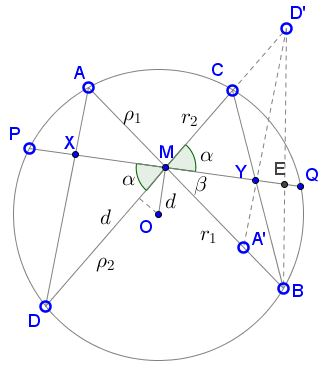The details can be found in a separate file.

### Proof 22

In this proof, Martin Celli exploits the fact that the two wings of a butterfly are similar amd finds an explicit form of the scale factor. The details are in a separate file.

### Proof 23

In this proof, Tran Quang Hung chases angles to find inscribed quadrilaterals and draws triangle midlines.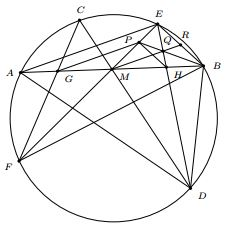The details are in a separate file.

### Proof 24

The proof by Stathis Koutras employs an elegant theorem of his applied in the following configuration.The details are in a separate file.

### Proof 25

Albrecht Hess gave a proof in complex numbers for a statement based on the original (see above) diagram. The proof appears in a separate page.

### Reference

1. H. S. M. Coxeter, S. L. Greitzer, Geometry Revisited, MAA, 1967
2. Alex D D Craik and John J O'Connor, Some unknown documents associated with William Wallace (1768-1843), BSHM Bulletin: Journal of the British Society for the History of Mathematics, 26:1, 17-28
3. S. L. Greitzer, Arbelos, v 5, ch 2, pp 38-39, MAA, 1991
4. R. Honsberger, The Butterfly Problem and Other Delicacies from the Noble Art of Euclidean Geometry I, TYCMJ, 14 (1983), pp. 2-7.
5. R. Honsberger, Mathematical Diamonds, MAA, 2003
6. V. V. Prasolov, Essays On Numbers And Figures, AMS, 2000
7. V. V. Prasolov, Problems in Planimetry, v 1, Nauka, Moscow, 1986, in Russian
8. V. V. Prasolov, Problems in Planimetry, v 2, Nauka, Moscow, 1986, in Russian
9. D. O. Shklyarsky, N. N. Chentsov, I. M. Yaglom, Selected Problems and Theorems of Elementary Mathematics, v 2, Moscow, 1952.### Butterfly Theorem and Variants•